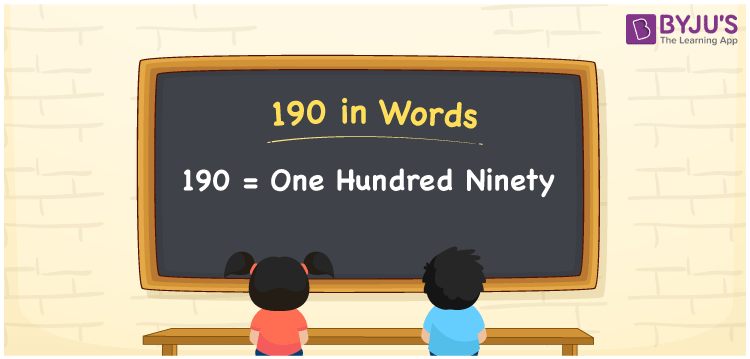# 190 in Words

190 in words is written as “One Hundred Ninety”. If you give 190 rupees to your friend, then you can say “I have given Rupees One Hundred Ninety to my friend”. In Mathematics, 190 is a natural number or an integer that is used to represent a value or a quantity. Learn to write the number 190 in words using the place value method and more about Numbers In Words at BYJU’S.

 190 in Words One Hundred Ninety One Hundred Ninety in Numbers 190

## 190 in English Words

190 in Words using English alphabets is expressed as given below:## How to Write 190 in Words?

190 in words is written based on the position of each digit in the given number. Since 190 is a three-digit number, thus the digits can be represented as:

 Hundreds Tens Ones 1 9 0

From the above table, we can see,

• 1 is at Hundreds
• 9 is at Tens
• 0 is at Ones

We need to read the number from right to left, in the table. Hence, it is spelt as One Hundred Ninety.

### Expanded Form of 190

We can write the expanded form of 190 as:

1 at Hundreds + 1 at Tens + 8 at Ones

= 1 × 100 + 9 × 10 + 0 × 1

= 100 + 90 + 0

= 100 + 90

= 190

= One Hundred Ninety

190 is a whole number that is succeeded by 189 and preceded by 191. Find more about the number 190 below:

• 190 in Words – One Hundred Ninety
• Is 190 an odd number? – No
• Is 190 an even number? – Yes
• Is 190 a perfect square number? – No
• Is 190 a perfect cube number? – No
• Is 190 a prime number? – No
• Is 190 a composite number? – Yes

## Frequently Asked Questions on 190 in words

Q1

### What is 190 in words?

190 in words is given by One Hundred Ninety.
Q2

### What is the rule to write 190 in words?

190 in words is written based on the place values of each digit.

1 → Hundreds

9 → Tens

0 → Ones

Thus, the number is read as One Hundred Ninety.

Q3

### What is the value of 190 + 100 in words?

190 + 100 = 290, i.e., Two Hundred Ninety, in words.